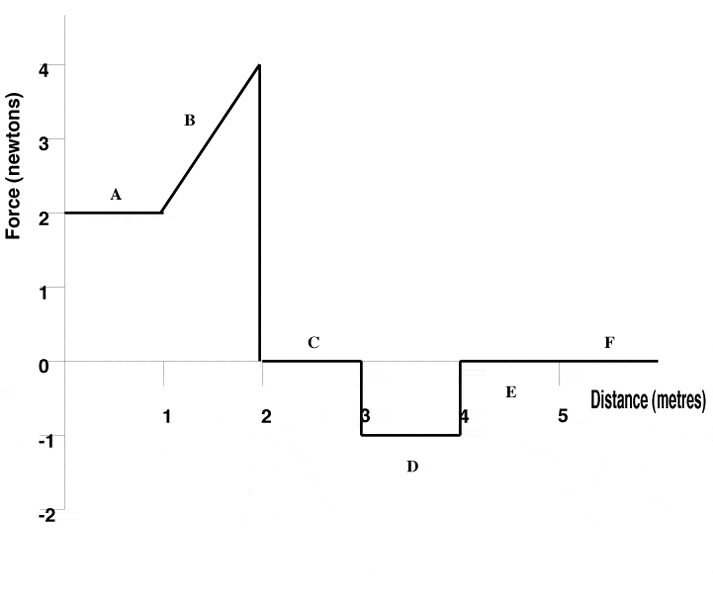# Kinetic Energy of body moving

## Homework Statement

The graph below shows the net force applied to a 2.5 kg body, initially at rest but free to move along a straight line on a horizontal frictionless surface. The force acts along the same straight line.After the body has moved 3 m, what is it's kinetic energy?

KE=1/2mv^2

## The Attempt at a Solution

I just don't understand how to figure out the velocity of the body given force versus distance. Can someone explain this?

Last edited:

Dick
Homework Helper
You haven't really stated a question. But the work done on the body by a force is the integral of F*dx. And the KE of the body is (1/2)*mv^2. And the change of the kinetic energy is the work done on the body. This is the same thing we were talking about last night. Except that was the simpler case where the force was a constant. Hence mgh. F=mg, distance=h.

My bad, the question is that after the body has moved 3 m, what is it's kinetic energy?

How do I figure out the velocity?

Dick
Homework Helper
Was it's initial kinetic energy zero? Then the integral of the force (area under the force curve)=KE.

So then I can just make squares and triangles and figure out the area right? So then I would get the KE is 4???

Dick
Homework Helper
You mean 4J, right? Always put units on things. But still not right. It would be 4J is the segment B were flat. It's not. Do make squares and triangles.

Last edited:
Yeah, I meant 4 Joules. But you can separate section A and part of B into a square and the area of that is 4 J. Then you can find the area of the triangle on part B which would be 1J and then subtract part C from those. Where am I going wrong?

Dick